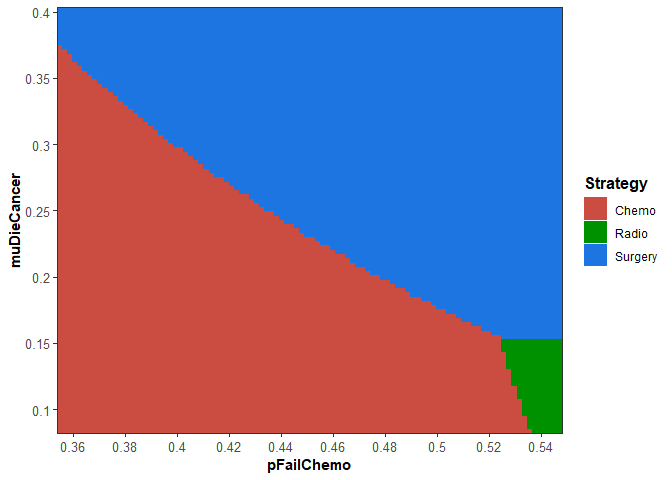# Overview

The probabilistic sensitivity analysis (PSA) object is a key part of the dampack package. In this vignette, we discuss the goals of a probabilistic sensitivity analysis, how to create the dampack PSA object from an existing PSA dataset, and what can be done once the PSA object is created in dampack.

dampack has additional functionality for generating a PSA dataset with a user-defined R-based decision model and specified parameter distributions. Those methods are covered in the psa_generation vignette (type vignette("psa_generation", package = "dampack") in the console after installing the dampack package). Even if you intend to generate your own PSA dataset using dampack, it is recommended that you continue reading this vignette (psa_analysis) first.

## Probability Sensitivity Analysis: An Introduction

The purpose of a PSA is to translate model parameter uncertainty into decision uncertainty, measured as the probability that a given strategy is optimal. In the context of cost-effectiveness analysis, this is the probability that a given strategy is cost-effective, defined as achieving the maximum health benefit at a cost per unit benefit gained less than a defined maximum willingness-to-pay (WTP) per unit benefit. The cost-effective strategy is equivalently defined as the strategy that maximizes the net monetary benefit (NMB) calculated as WTP * Benefit - Cost, with an appropriately set WTP. Thus, in the PSA examples, we will generally use NMB as the outcome of each strategy.

Parameter uncertainty is reflected in probability distributions defined for each model parameter. Common distributions include the normal, beta, gamma, and log-normal distributions. To generate a single PSA sample, a value is randomly drawn for each parameter from its respective distribution. The model is then run for that set of parameter values to calculate the resulting outcomes of interest for each strategy. A large number of PSA samples, say 10,000, are generated in this way, with each iteration using one set of values from the distributions of the parameters and each iteration yielding one set of outcome values. The resulting distribution of outcome values across the PSA samples translates into decision uncertainty when certain strategies are favored in some samples but not in others.

For a more in-depth discussion of cost-effectiveness analysis and the role of PSA, we recommend the following readings: - Alarid-Escudero, Fernando, Eva A. Enns, Karen M. Kuntz, Tzeyu L. Michaud, and Hawre Jalal. “Time traveling is just too dangerous” but some methods are worth revisiting: the advantages of expected loss curves over cost-effectiveness acceptability curves and frontier. Value in Health 2019; 22(5):611-618. - Cost-Effectiveness in Health and Medicine, 2nd edition, eds: Neumann PJ, Ganiats TG, Russell LB, Sanders GD, Siegel JE. Oxford University Press, 2016.

## PSA in dampack

The PSA object is created using the function make_psa_obj. We can see the types of data we need by examining what arguments this function takes. To see more information, you can write ?make_psa_obj in the R console. As the arguments (and the help text) show, we need the cost, effectiveness, strategies (optional), and currency (also optional).

There is example data provided within dampack that allows us to illustrate the required structure of each of these parts of a PSA.

library(dampack)
data("example_psa")

We can see the structure of the object with str():

str(example_psa)
#> List of 5
#>  $strategies : chr [1:3] "Chemo" "Radio" "Surgery" #>$ wtp          : num [1:15] 1000 11000 21000 31000 41000 51000 61000 71000 81000 91000 ...
#>  $cost :'data.frame': 10000 obs. of 3 variables: #> ..$ Chemo_Cost: num [1:10000] 33683 30131 26839 28671 31540 ...
#>   ..$Radio_Cost: num [1:10000] 8942 19705 13250 21505 13163 ... #> ..$ Surg_Cost : num [1:10000] 29706 28565 22430 28498 12835 ...
#>  $effectiveness:'data.frame': 10000 obs. of 3 variables: #> ..$ Chemo_Eff: num [1:10000] 12.73 9.85 10.05 11.68 10.76 ...
#>   ..$Radio_Eff: num [1:10000] 12.13 9.86 10.01 11.01 10.75 ... #> ..$ Surg_Eff : num [1:10000] 11.26 10.19 9.83 10.86 10.57 ...
#>  $parameters :'data.frame': 10000 obs. of 8 variables: #> ..$ pFailChemo : num [1:10000] 0.391 0.425 0.458 0.474 0.484 ...
#>   ..$pFailRadio : num [1:10000] 0.501 0.418 0.455 0.557 0.47 ... #> ..$ pFailSurg  : num [1:10000] 0.1264 0.036 0.0433 0.0487 0.0531 ...
#>   ..$pDieSurg : num [1:10000] 0.0989 0.0876 0.1389 0.1426 0.1178 ... #> ..$ muDieCancer: num [1:10000] 0.113 0.285 0.257 0.15 0.201 ...
#>   ..$cChemo : num [1:10000] 22569 21018 17874 17687 19810 ... #> ..$ cRadio     : num [1:10000] 5307 13925 8927 12215 8492 ...
#>   ..$cSurg : num [1:10000] 29706 28565 22430 28498 12835 ... We can ignore the wtp part for now. The other parts of the example_psa object are: • strategies: a list of three strategy names example_psa$strategies
#>  "Chemo"   "Radio"   "Surgery"
• cost: a data frame of the cost of carrying out each strategy. The columns are the strategies and the rows are the PSA samples (of which there were 10,000).
head(example_psa$cost) #> Chemo_Cost Radio_Cost Surg_Cost #> 1 33682.76 8942.488 29705.66 #> 2 30130.87 19704.842 28565.46 #> 3 26838.94 13249.548 22429.61 #> 4 28671.30 21505.019 28498.20 #> 5 31540.26 13163.105 12835.14 #> 6 32618.48 17059.544 42954.16 • effectiveness: a data frame of the effects of carrying out each strategy, with the same structure as costs head(example_psa$effectiveness)
#> 1  12.72801 12.130626 11.263131
#> 2   9.85347  9.855394 10.193810
#> 3  10.05273 10.011711  9.831964
#> 4  11.67804 11.013624 10.856675
#> 5  10.75947 10.753719 10.574672
#> 6  12.30980 11.816024 11.984267

Note that the columns have names that suggest the strategy and the outcome type in the example_psa. When using the make_psa_obj() function, it is not actually necessary for the column names in the cost and effectiveness data frame inputs to follow this convention; however, it is critical that the vector of strategies (example_psa$strategies) and the columns of the cost and effectiveness data frames be in the same order. Now, let’s make the PSA object and look at its structure: psa_obj <- make_psa_obj(cost = example_psa$cost,
effectiveness = example_psa$effectiveness, parameters = example_psa$parameters,
strategies = example_psa$strategies, currency = "$")
str(psa_obj)
#> List of 9
#>  $n_strategies : int 3 #>$ strategies   : chr [1:3] "Chemo" "Radio" "Surgery"
#>  $n_sim : int 10000 #>$ cost         :'data.frame':   10000 obs. of  3 variables:
#>   ..$Chemo : num [1:10000] 33683 30131 26839 28671 31540 ... #> ..$ Radio  : num [1:10000] 8942 19705 13250 21505 13163 ...
#>   ..$Surgery: num [1:10000] 29706 28565 22430 28498 12835 ... #>$ effectiveness:'data.frame':   10000 obs. of  3 variables:
#>   ..$Chemo : num [1:10000] 12.73 9.85 10.05 11.68 10.76 ... #> ..$ Radio  : num [1:10000] 12.13 9.86 10.01 11.01 10.75 ...
#>   ..$Surgery: num [1:10000] 11.26 10.19 9.83 10.86 10.57 ... #>$ other_outcome: NULL
#>  $parameters :'data.frame': 10000 obs. of 8 variables: #> ..$ pFailChemo : num [1:10000] 0.391 0.425 0.458 0.474 0.484 ...
#>   ..$pFailRadio : num [1:10000] 0.501 0.418 0.455 0.557 0.47 ... #> ..$ pFailSurg  : num [1:10000] 0.1264 0.036 0.0433 0.0487 0.0531 ...
#>   ..$pDieSurg : num [1:10000] 0.0989 0.0876 0.1389 0.1426 0.1178 ... #> ..$ muDieCancer: num [1:10000] 0.113 0.285 0.257 0.15 0.201 ...
#>   ..$cChemo : num [1:10000] 22569 21018 17874 17687 19810 ... #> ..$ cRadio     : num [1:10000] 5307 13925 8927 12215 8492 ...
#>   ..$cSurg : num [1:10000] 29706 28565 22430 28498 12835 ... #>$ parnames     : chr [1:8] "pFailChemo" "pFailRadio" "pFailSurg" "pDieSurg" ...
#>  $currency : chr "$"
#>  - attr(*, "class")= chr [1:2] "psa" "sa"

The psa object has its own specialized plotting functionality, and the input options for plot.psa can be displayed by typing ?plot.psa in the console. Each dot in the plot represents the the cost and effectiveness of a specific strategy for a single sample of the PSA. The ellipses (shown by default) helps to visualize the clustering of each strategy on the cost-effectiveness plane.

plot(psa_obj)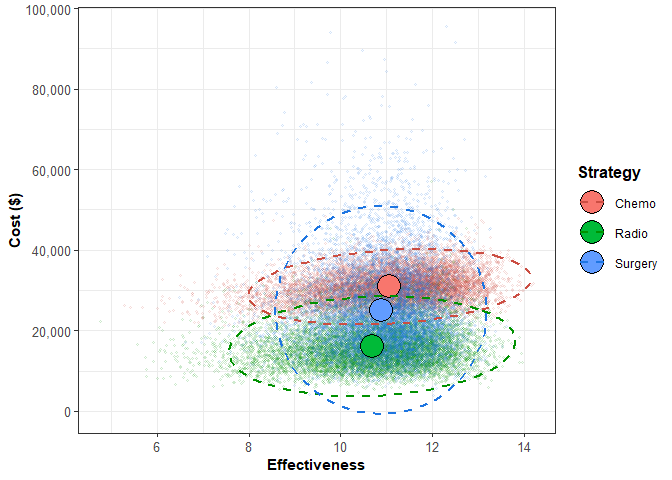When you call summary() on a psa object, a data.frame is returned that displays the mean cost and mean effect across all of the PSA samples for each strategy. If calc_sds is set to TRUE, then the standard deviations for these outcomes are displayed as well.

psa_sum <- summary(psa_obj,
calc_sds = TRUE)
psa_sum
#>   Strategy meanCost meanEffect    sdCost  sdEffect
#> 1    Chemo 30900.80   11.06869  3841.172 1.2599541
#> 2    Radio 16128.88   10.68492  5073.654 1.2768415
#> 3  Surgery 25110.22   10.88120 10523.753 0.9418639

For more information about how to use dampack to calculate, visualize, and analyze the ICERs for the different strategies compared in a PSA, please see the basic_cea vignette.

icers <- calculate_icers(cost = psa_sum$meanCost, effect = psa_sum$meanEffect,
strategies = psa_sum$Strategy) plot(icers)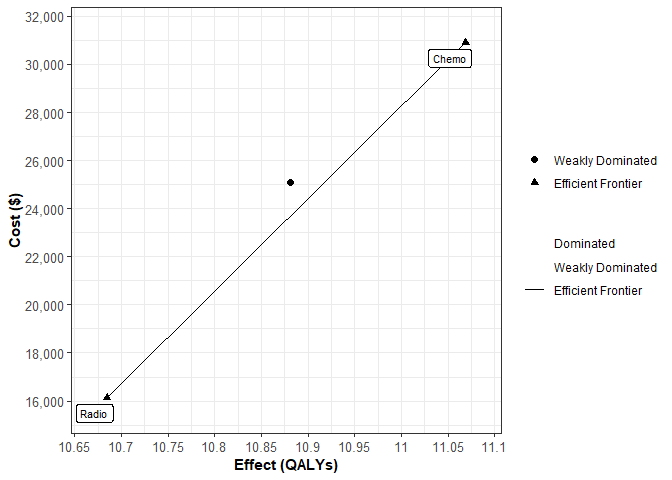## Cost-effectiveness Acceptability Curve A strategy’s cost-effectiveness is sometimes discussed in terms of either net health benefit or net monetary benefit. To calculate a strategy’s net health benefit, the cost associated with the strategy is divided by the willingness-to-pay threshold (WTP) and added to the QALYs gained, and to calculate a strategy’s net monetary benefit, the number of QALYs gained is multiplied by the WTP threshold and added to the cost. Describing strategies in terms of net health benefit or net monetary benefit is convenient because it allows one to compare the cost-effectiveness of the strategies using a single metric, but these comparisons are sometimes very sensitive to the exact WTP threshold that is used to convert QALYs to currency, or vice versa. The degree to which the choice of willingness-to-pay threshold influences the decision can be analyzed using a cost-effectiveness acceptability curve. The acceptability curves plot the relative frequency or probability that each strategy is cost-effective compared to the alternatives for varying values for society’s willingness-to-pay. The ceac() function (ceac stands for cost-effectiveness acceptability curve) takes a psa object and a numeric vector of willingness to pay thresholds to create a ceac object, which is essentially a data.frame with special plotting functionality. The proportion column of the ceac object is the proportion of PSA samples in which that particular strategy had the maximum benefit at the corresponding WTP. The On_Frontier column indicates whether a strategy was on the cost-effectiveness acceptability frontier at the corresponding WTP. A strategy is on the cost-effectiveness acceptability frontier if it maximizes expected benefit on average across all samples of the PSA at the WTP being considered. Note that this designation is fundamentally different than identifying the strategy that has the highest probability of being cost-effective across all samples of the PSA. ceac_obj <- ceac(wtp = example_psa$wtp,
psa = psa_obj)
#>     WTP Strategy Proportion On_Frontier
#> 1  1000    Chemo     0.0073       FALSE
#> 2  1000    Radio     0.7799        TRUE
#> 3  1000  Surgery     0.2128       FALSE
#> 4 11000    Chemo     0.0579       FALSE
#> 5 11000    Radio     0.6379        TRUE
#> 6 11000  Surgery     0.3042       FALSE

Using the summary() function on a ceac object yields a data.frame that reports the strategies that are on the cost-effectiveness acceptability frontier along with the ranges of WTP values for which these optimal strategies are most cost-effective.

summary(ceac_obj)
#>   range_min range_max cost_eff_strat
#> 2     41000    141000          Chemo

Plotting a ceac object yields a ggplot2 graph showing the cost-effectiveness acceptability curves for all strategies where the y-axis indicates the probability that each strategy is cost-effective and the x-axis indicates the WTP threshold. By default, the strategies on the cost-effectiveness acceptability frontier are marked with black boxes, and the exact values calculated within the ceac object at each provided WTP threshold are marked with points. For more plotting details, see ?plot.ceac.

plot(ceac_obj,
frontier = TRUE,
points = TRUE)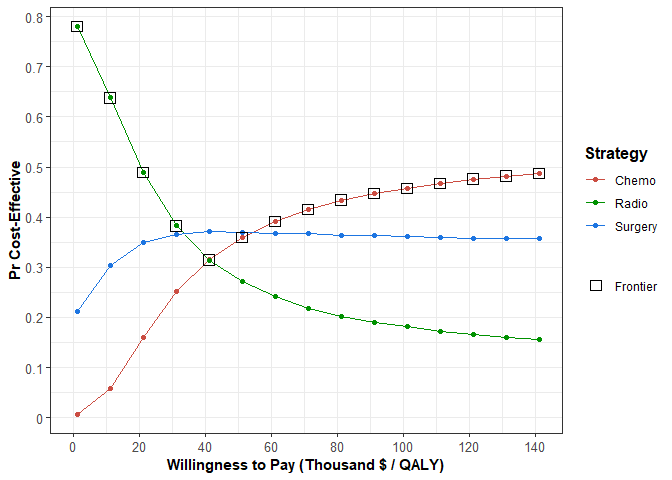## Expected Loss Curve

Alternatively, the degree to which cost-effectiveness varies with respect to the WTP threshold can be visualized using an expected loss curve (ELC). Expected loss is a quantification of the consequences of choosing a suboptimal strategy in terms of expected foregone benefits. The strategy that minimizes the expected loss at a given WTP value is the optimal decision given current information, and consequently the graph’s relationship to the cost-effectiveness acceptability frontier is somewhat more intutive than for CEACs. For an in-depth discussion on the advantages of ELCs in cost-effectiveness analysis, please refer to:

Alarid-Escudero F, Enns EA, Kuntz KM, Michaud TL, Jalal H. “Time Traveling Is Just Too Dangerous” But Some Methods Are Worth Revisiting: The Advantages of Expected Loss Curves Over Cost-Effectiveness Acceptability Curves and Frontier. Value Health. 2019;22(5):611-618.

el <- calc_exp_loss(wtp = example_psa\$wtp,
psa = psa_obj)
#>     WTP Strategy Expected_Loss On_Frontier
#> 1  1000    Chemo     15472.987       FALSE
#> 2  1000    Radio      1084.833        TRUE
#> 3  1000  Surgery      9869.887       FALSE
#> 4 11000    Chemo     13163.617       FALSE
#> 5 11000    Radio      2613.132        TRUE
#> 6 11000  Surgery      9435.351       FALSE
plot(el,
n_x_ticks = 8,
n_y_ticks = 6)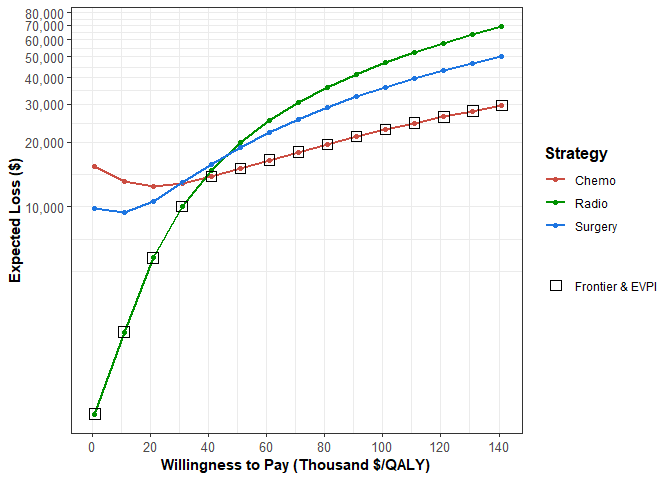## One-way Sensitivity Analysis

A one-way sensitivity analysis (OWSA) illustrates how the expected outcome of a decision model changes as function of a single input parameter in the PSA. Knowing that the parameters in a decision model can interact in complex and nonlinear ways to influence outcomes, the characterization of the role of a single parameter is not straightforward. To disentangle the effect of the parameter of interest, a regression model, or ‘metamodel’, is fit using the entire PSA that treats the specified outcome (Net-monetary benefit, QALYs, etc) as the dependent variable and the parameter as the independent variable. By omitting the other input parameters in the PSA in the regression model, the variation in the outcome that cannot be directly attributed to variation in the parameter of interest is treated as random statistical error (even if the model is purely deterministic). Consequently, any predicted outcome value derived from the metamodel is essentially conditional on the average value of all the other PSA parameters. Separate models are produced for each strategy included in the strategies argument of the owsa() function, and these models are used to predict outcome values over a range values of the parameter of interest.

The first input of the owsa() function is sa_obj, which stands for “sensitivity analysis object”, because the owsa() function can also be used in the context of a determinstic sensitivity analysis on a dsa object. For an illustration of this functionality, enter vignette("dsa_visualization", package = "dampack") in the console. The params argument allows the user to execute a series of separate one-way sensitivity analyses with a single function call by specifying a vector of different parameters of interest. By default, the regression model used to quantify the effect of the parameter of interest on the expected outcome value is a second order polynomial linear model. The order of the polynomial in the regression model can be altered by changing the argument poly.order. A higher value of poly.order will yield a more flexible metamodel, which can potentially lead to overfitting.

o <- owsa(psa_obj)

The output of the owsa() function has customized plotting functionality that can be explored by typing ?plot.owsa in the console. Like all other plots produced by dampack, plots derived from the owsa object are ggplot objects and can be readily customized.

plot(o,
n_x_ticks = 2)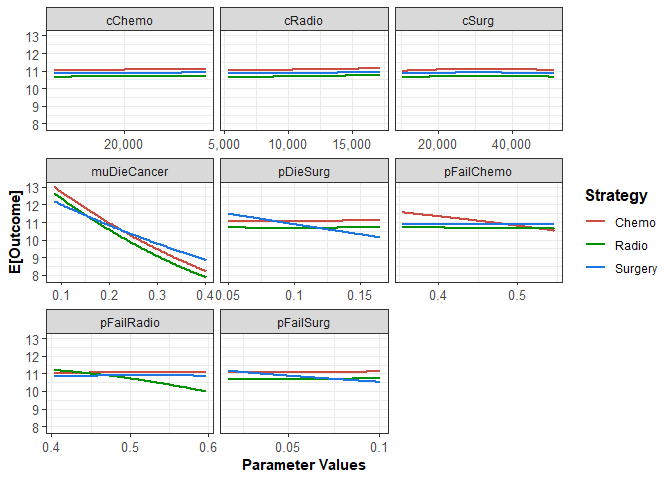Using the owsa() function on a PSA object will produce an owsa class object, and this object is compatible with two special plotting functions in addition to plot.owsa(). The first of these plotting functions is owsa_tornado, which produces a tornado plot. A tornado plot is a visual aid used to identify which parameters are driving most of the variation in a specified model outcome. In addition, the plot shows whether high or low expected outcome values result from parameter values that are above or below the median value of the parameter in question (indicated by the fill color corresponding to “Parameter Level High/Low”. For example, the tornado plot below tells us that the parameter "muDieCancer" has the most leverage in affecting the effectiveness model outcome, and that values below the median value of "muDieCancer" in the one-way sensitivity analysis are associated with higher expected effectiveness outcomes.

It is important to note that some important information is obscured by tornado plots and caution should be exercised when interpreting it. As the parameter of interest varies across its range in the one-way sensitivity, the strategy that maximizes the outcome of interest can also change across this range. The plot is not showing how the expected outcome changes for a single strategy, but how the expected outcome of the optimal strategy changes. The designation of which strategy is optimal is liable to alternate over the range of the parameter of interest, and this is hidden in a tornado plot.

owsa_tornado(o)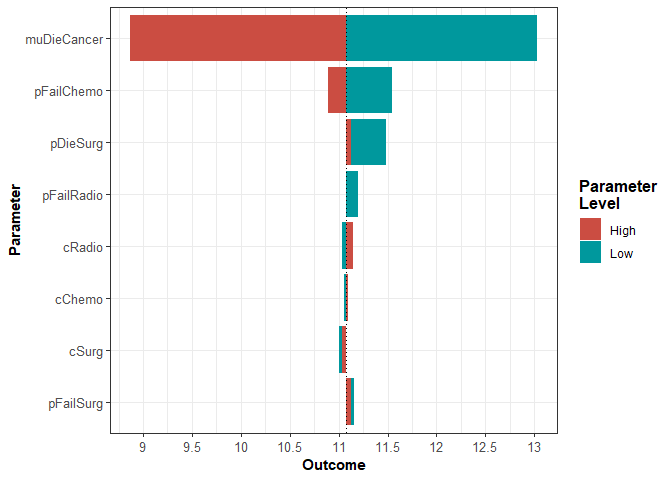For owsa objects that contain many parameters that have minimal effect on the parameter of interest, you may want to consider producing a plot that highlights only the most influential parameters. Using the min_rel_diff argument, you can instruct owsa_tornado to exclude all parameters that fail to produce a relative change in the outcome below a specific fraction.

owsa_tornado(o,
min_rel_diff = 0.05)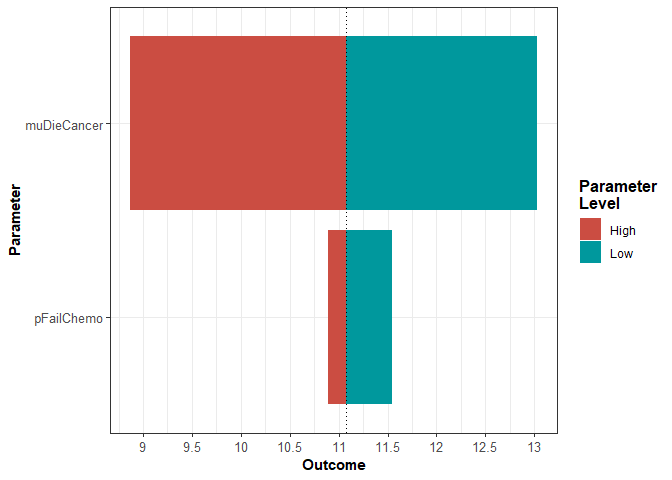In order to attain the data.frame used to produce the tornado plot, use the return argument to change the type of object returned by the owsa_tornado function.

owsa_tornado(o,
return = "data")
#> # A tibble: 8 x 7
#> # Groups:   parameter 
#>   parameter   param_val.low outcome_val.low param_val.high outcome_val.high
#>   <chr>               <dbl>           <dbl>          <dbl>            <dbl>
#> 1 muDieCancer        0.0837            13.0          0.402             8.87
#> 2 pFailChemo         0.355             11.6          0.547            10.9
#> 3 pDieSurg           0.0498            11.5          0.165            11.1
#> 4 pFailRadio         0.403             11.2          0.597            11.1
#> 5 cRadio          5315.                11.0      17203.               11.1
#> 6 cChemo         16291.                11.1      24289.               11.1
#> 7 cSurg          10474.                11.0      51427.               11.0
#> 8 pFailSurg          0.0168            11.2          0.100            11.1
#> # ... with 2 more variables: abs_diff <dbl>, rel_diff <dbl>

### Optimal Strategy Plot

The second special plotting function designed for the visualization of the owsa object is owsa_opt_strat, which directly addresses the crucial information that is missing from the tornado plot. The output of owsa_opt_strat allows us to see how the strategy that maximizes the expectation of the outcome of interest changes as a function of each parameter of interest.

owsa_opt_strat(o,
n_x_ticks = 5)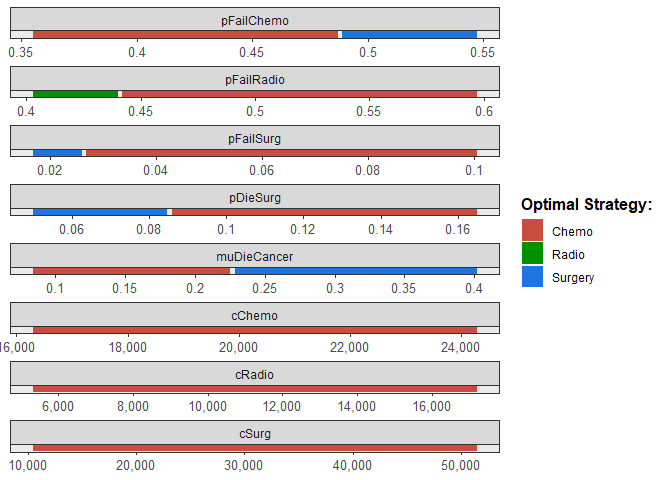Like owsa_tornado(), the return argument in owsa_opt_strat() allows the user to access a tidy data.frame that contains the exact values used to produce the plot.

owsa_opt_strat(o,
return = "data")
#> # A tibble: 13 x 4
#>    parameter   strategy       pmin       pmax
#>    <fct>       <fct>         <dbl>      <dbl>
#>  1 cChemo      Chemo    16291.     24289.
#>  2 cRadio      Chemo     5315.     17203.
#>  3 cSurg       Chemo    10474.     51427.
#>  4 muDieCancer Chemo        0.0837     0.225
#>  5 muDieCancer Surgery      0.228      0.402
#>  6 pDieSurg    Chemo        0.0859     0.165
#>  7 pDieSurg    Surgery      0.0498     0.0847
#>  8 pFailChemo  Chemo        0.355      0.487
#>  9 pFailChemo  Surgery      0.489      0.547
#> 10 pFailRadio  Chemo        0.442      0.597
#> 12 pFailSurg   Chemo        0.0269     0.100
#> 13 pFailSurg   Surgery      0.0168     0.0261

## Two-way Sensitivty Analysis

A two-way sensitivity analysis (TWSA) illustrates how the expected outcome of a decision-analytic model changes as function of two input parameters in the PSA. Just as a regression metamodel was used to isolate the effect of a single parameter in dampack’s owsa function, twsa() uses a polynomial regression metamodel that includes both of the specified parameters to predict the expected outcome as a function of the two parameters. This regression metamodel includes polynomial terms up to and including the degree of the poly.order argument for both parameters, as well as all interaction terms between these polynomial terms.

tw <- twsa(psa_obj,
param1 = "pFailChemo",
param2 = "muDieCancer")

The default plot of a twsa object (see ?plot.twsa) shows the range of the two parameters of interest on the axes, with each region color coded according to which strategy maximizes the expected outcome of interest.

plot(tw)